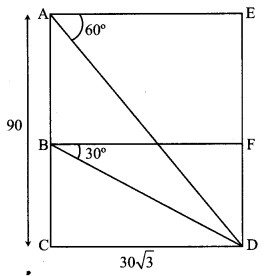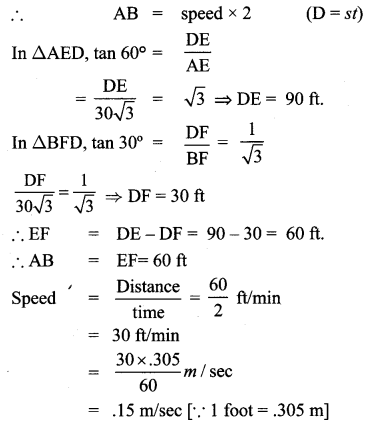## Tamilnadu Samacheer Kalvi 10th Maths Solutions Chapter 6 Trigonometry Ex 6.3

10th Maths Exercise 6.3 Samacheer Kalvi Question 1.
From the top of a rock 50$$\sqrt{3}$$ m high, the angle of depression of a car on the ground is observed to be 30°. Find the distance of the car from the rock.
Solution: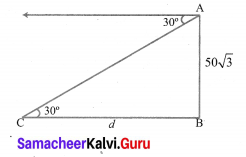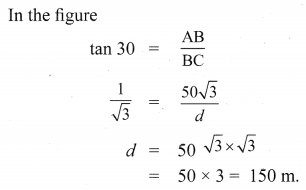Ex 6.3 Class 10 Samacheer Question 2.
The horizontal distance between two buildings is 70 m. The angle of depression of the top of the first building when seen from the top of the second building is 45°. If the height of the second building is 120 m, find the height of the first building.
Solution: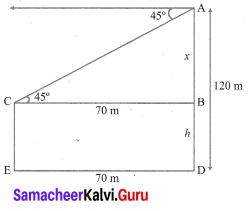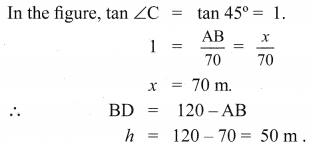∴ The height of the first building is 50m.

10th Maths 6.3 Question 3.
From the top of the tower 60 m high the angles of depression of the top and bottom of a vertical lamp post are observed to be 38° and 60° respectively. Find the height of the lamp post, (tan 38° = 0.7813, $$\sqrt{3}$$ = 1.732)
Solution: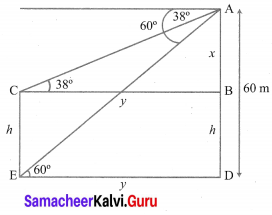From the figure,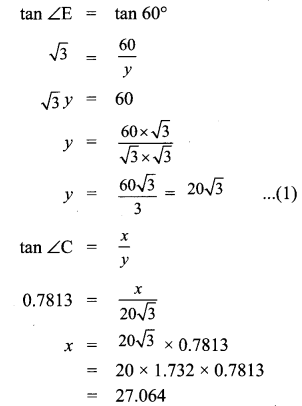∴ The height of the lamp post = CE
CE = BD = 60 – 27.064 = 32.93 m.

10th Maths Trigonometry Exercise 6.3 Question 4.
An aeroplane at an altitude of 1800 m finds that two boats are sailing towards it in the same direction. The angles of depression of the boats as observed from the aeroplane are 60° and 30° respectively. Find the distance between the two boats. ($$\sqrt{3}$$ = 1.732)
Solution: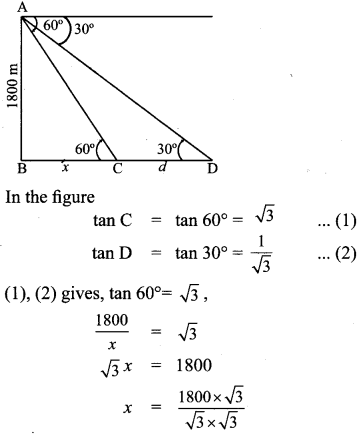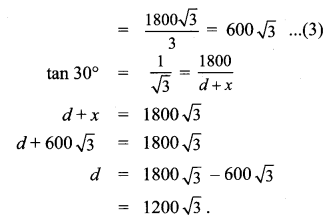Distance between the boats = 1200$$\sqrt{3}$$ m
= 2078.4 m

10th Maths Exercise 6.3 Question 5.
From the top of a lighthouse, the angle of depression of two ships on the opposite sides of it are observed to be 30° and 60°. If the height of the lighthouse is h meters and the line joining the ships passes through the foot of the lighthouse, show that the distance between the ships is $$\frac{4 h}{\sqrt{3}}$$ m.
Solution: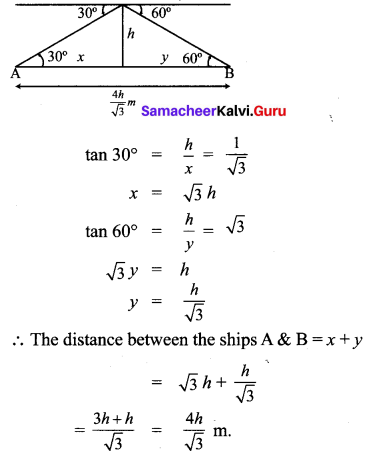It is proved.

10th Maths Exercise 6.4 Samacheer Kalvi Question 6.
A lift in a building of height 90 feet with transparent glass walls is descending from the top of the building. At the top of the building, the angle of depression to a fountain in the garden is 60°. Two minutes later, the angle of depression reduces to 30°. If the fountain is $$30 \sqrt{3}$$ feet from the entrance of the lift, find the speed of the lift which is descending.
Solution: